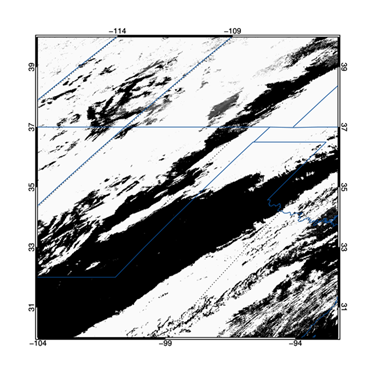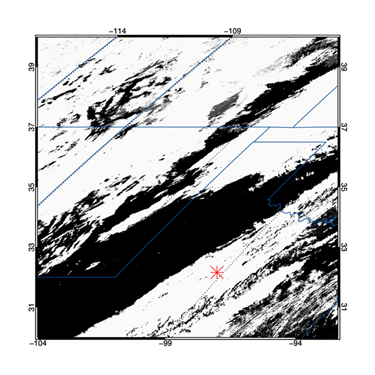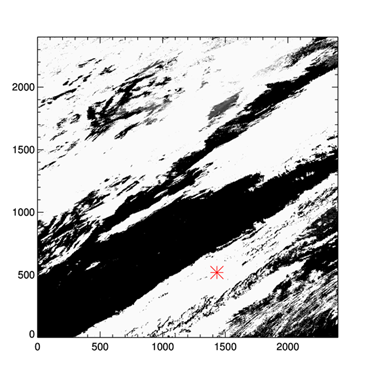Identifing the Pixel Location of a Latitude/Longitude PointQUESTION: I have a MODIS image in a GeoTiff file format. I would like to identify the pixel location in this image that is associated with a particular latitute and longitude. Can you show me how to do this with IDL? The longitude I want is -99.25 degrees west and the latitude is 32.16 degrees north.ANSWER: It will be helpful, I think, to see this image. MODIS images like this use a Sinusoidal grid. This particular image, you can see from the filename, is in grid cell 9 in the horizontal direction and grid cell 5 in the vertical direction. You can see from this MODIS map that the image covers a portion of the southern United States.

Here is the code I would use to read the file, create a map coordinate system, and display the image. Note, I am using Coyote Library routines to read, display, and navigate the image.

file = "MOD09GA.A2008006.h09v05.005.tif"
mapCoord = cgGeoMap(file, Image=geoImage)
pos = [0.1, 0.1, 0.9, 0.9]
cgDisplay, 800, 800
cgImage, geoImage, Position=pos
mapCoord -> SetProperty, Position=pos
cgMap_Grid, /CGGrid, /Box_Axes, Map=mapCoord
cgMap_Continents, /USA, Map=mapCoord, Color='blu7'

You see the result in the figure below.The image file with a map coordinate system established and the image annotated.

The next step is to make coordinate vectors that discribe the location, in projected meter space, of each image pixel. This is easily done by obtaining the image data range from the map coordinate object, like this. Note that the coordinate vectors contain one more pixel value than the size of the image in that direction. This is because the data ranges go from the outside boundaries of the image pixels on the left (or bottom) of the image to the outside boundaries of the image pixels on the right (or top) of the image. The coordinate vectors do not specify the locations at the center of the pixels. Rather, they specify the locations of the pixel edges.

mapCoord -> GetProperty, XRANGE=xr, YRANGE=yr
s = Size(img, /Dimensions)
xvec = cgScaleVector(Findgen(s+1), xr, xr)
yvec = cgScaleVector(Findgen(s+1), yr, yr)

Next, we identify the latitude and longitude we want to find in the image.

longitude = -99.25
latitude = 32.16

Since our coordinate vectors are in projected meter space, we need to forward project the latitude and longitude values into that space. We do so and plot the point on our map with this code.

xy = mapCoord -> Forward(longitude, latitude)
cgPlots, xy, xy, PSYM=2, SymSize=3, Color='red'

Finally, we simply find this location in our coordinate vectors and print the image pixel location. Note that we are not checking that the latitude and longitude is actually inside our image. We should be, since we will get incorrect results if this is the case.

xpixel = Value_Locate(xvec, xy)
ypixel = Value_Locate(yvec, xy)
Print, xpixel, ypixel
1433         519

Here we see that this latitude/longitude location corresponds to pixel value [1433,519] in the image. To see that this is the case, we can display the image with its pixel values and show that these two images show the locations in the same place.

cgDisplay, 800, 800, Wid=1
cgImage, geoImage, Position=pos, /Axes
cgPlots, xpixel, ypixel, PSYM=2, SymSize=3, Color='red'

The figure below has the two displays side-by-side.The image with the lat/lon location in map coordinates on the left and in pixel coordinates on the right.

You can find the code I used to produce the figures here.